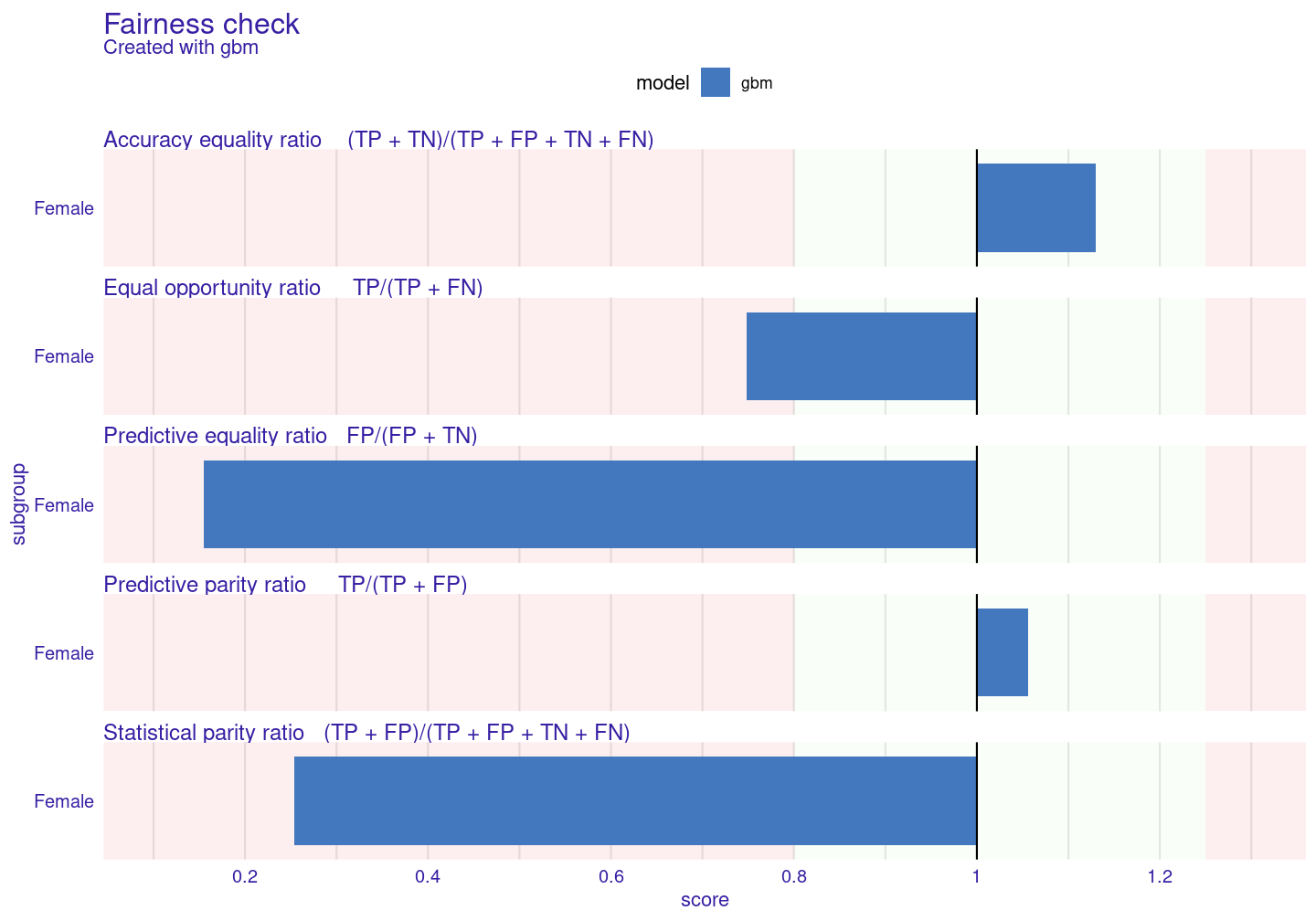# 1 Model, explanation & bias

In this tutorial you will learn how to tackle bias using the bias mitigation techniques supported by `fairmodels`. As always we will start from the data.

``````library(fairmodels)

``# for this vignette data will be truncated``

We will use adult data to predict whether certain person has yearly salary exceeding 50 000 or not. Our protected variable will be sex. For this tutorial we will be using `gbm` and of course we will explain it with `DALEX`.

``````library(gbm)
library(DALEX)

# making model
set.seed(1)
gbm_model <-gbm(salary ~. , data = adult, distribution = "bernoulli")

# making explainer
gbm_explainer <- explain(gbm_model,
colorize = FALSE)``````
``````#> Preparation of a new explainer is initiated
#>   -> model label       :  gbm  (  default  )
#>   -> data              :  32561  rows  13  cols
#>   -> target variable   :  32561  values
#>   -> predict function  :  yhat.gbm  will be used (  default  )
#>   -> predicted values  :  numerical, min =  0.0101498 , mean =  0.2409542 , max =  0.9864558
#>   -> model_info        :  package gbm , ver. 2.1.8 , task classification (  default  )
#>   -> residual function :  difference between y and yhat (  default  )
#>   -> residuals         :  numerical, min =  -0.9790795 , mean =  -0.0001445991 , max =  0.9864904
#>   A new explainer has been created!``````
``model_performance(gbm_explainer)``
``````#> Measures for:  classification
#> recall     : 0.5353909
#> precision  : 0.7999238
#> f1         : 0.6414547
#> accuracy   : 0.8558705
#> auc        : 0.9093789
#>
#> Residuals:
#>          0%         10%         20%         30%         40%         50%
#> -0.97907954 -0.31512711 -0.20921106 -0.12255213 -0.06480941 -0.04122486
#>         60%         70%         80%         90%        100%
#> -0.02832005 -0.01740186  0.13344943  0.53676046  0.98649038``````

Our model has around 86% accuracy. And how about bias? Sex is our protected variable and we should suspect that men will be more frequently assigned better annual income.

``````fobject <- fairness_check(gbm_explainer,
protected  = protected,
privileged = "Male",
colorize = FALSE)``````
``````#> Creating fairness object
#> -> Privileged subgroup       : character ( Ok  )
#> -> Protected variable        : factor ( Ok  )
#> -> Cutoff values for explainers  : 0.5 ( for all subgroups )
#> -> Fairness objects      : 0 objects
#> -> Checking explainers       : 1 in total (  compatible  )
#> -> Metric calculation        : 12/12 metrics calculated for all models
#>  Fairness object created succesfully``````
``print(fobject, colorize = FALSE)``
``````#>
#> Fairness check for models: gbm
#>
#> gbm passes 2/5 metrics
#> Total loss:  2.028729``````

Our model passes only few metrics, how big is the bias?

``plot(fobject)``The biggest bias is in `Statistical parity loss` metric. It is metric that is frequently look at because it gives answer how much difference is there in positive label rates in model within protected variable. Let’s say that it will be metric that we will try to mitigate.

# 2 Bias mitigation strategies

## 2.1 Pre-processing techniques

Pre-processing techniques focus on changing data before model is trained. This reduces bias in data.

### 2.1.1 Distribution changing

Firs technique you will learn about is `disparate_impact_remover`. It is somehow limited as it works on ordinal, numeric data. This technique returns “fixed” data frame. Through parameter `lambda` we can manipulate with how much the distribution will be fixed. `lambda = 1` (default) will return data with identical distributions for all levels of protected variable whereas `lambda = 0` will barely change anything. We will transform a few features.

``````data_fixed <- disparate_impact_remover(data = adult, protected = protected,
features_to_transform = c("age", "hours_per_week",
"capital_loss",
"capital_gain"))

set.seed(1)
gbm_model     <- gbm(salary ~. , data = data_fixed, distribution = "bernoulli")
gbm_explainer_dir <- explain(gbm_model,
data = data_fixed[,-1],
``````fobject <- fairness_check(gbm_explainer, gbm_explainer_dir,